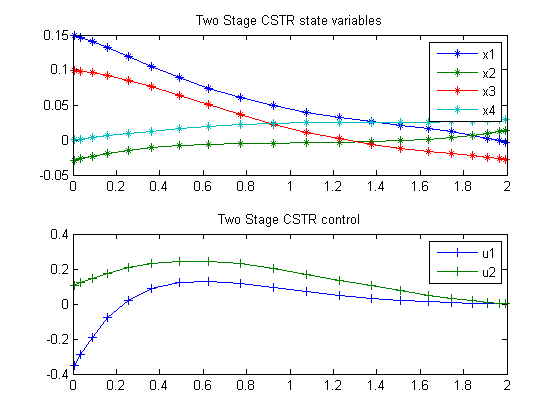123  Two Stage CSTR

ITERATIVE DYNAMIC PROGRAMMING, REIN LUUS

Section 6.3.1 Nonlinear two-stage CSTR system

CHAPMAN & HALL/CRC Monographs and Surveys in Pure and Applied Mathematics

123.1  Problem Description

The system consists of a series of two CSTRs, where there is a transportation delay tau = 0.1 from the first tank to the second. A truncated Taylor series expansion for the time delay.

Find u over t in [0; 2 ] to minimize

 J = x5(tF)

subject to:

 dx1 dt
= f1
 dx2 dt
= f2
 dx3 dt
= x1 − x3 − tau*f1 − R2 + 0.25
 dx4 dt
= x2 − 2*x4 − u2*(x4 + 0.25) − tau*f2 + R2 − 0.25
 dx5 dt
= x12x22 + x32 + x42 + 0.1*(u12 + u22

 f1 = 0.5 − x1 − R1
 f2 = −2*(x2 + 0.25) − u1*(x2 + 0.25) + R1
R1 = (x1 + 0.5)*exp(25*
 x2 x2 + 2
R2 = (x3 + 0.25)*exp(25*
 x4 x4 + 2

The state variables x1 and x3 are normalized concentration variables in tanks 1 and 2, respectively, and x2 and x4 are normalized temperature variables in tanks 1 and 2, respectively. The variable x5 is introduced to provide the performance index to be minimized.

The initial condition are:

 x(0) = [0.15  −0.03  0.10  0  0]
 −0.5 <= u1 <= 0.5
 −0.5 <= u2 <= 0.5

Reference: 

123.2  Problem setup

toms t
p = tomPhase('p', t, 0, 2, 20);
setPhase(p);

tomStates x1 x2 x3 x4 x5
tomControls u1 u2

xi = [0.15;-0.03;0.10;0;0];

% Initial guess
x0 = {icollocate({x1 == xi(1); x2 == xi(2)
x3 == xi(3); x4 == xi(4); x5 == xi(5)})
collocate({u1 == 0; u2 == 0})};

% Box constraints
cbox = collocate({-0.5 <= u1 <= 0.5
-0.5 <= u2 <= 0.5});

% Boundary constraints
cbnd = initial({x1 == xi(1); x2 == xi(2)
x3 == xi(3); x4 == xi(4); x5 == xi(5)});

% ODEs and path constraints
R1 = (x1 + 0.5).*exp(25*x2./(x2 + 2));
R2 = (x3 + 0.25).*exp(25*x4./(x4 + 2));
f1 = 0.5 - x1 - R1;
f2 = -2*(x2 + 0.25) - u1.*(x2 + 0.25) + R1;
tau = 0.1;
ceq = collocate({
dot(x1) == f1; dot(x2) == f2
dot(x3) == x1-x3-tau*f1-R2+0.25
dot(x4) == x2-2*x4-u2.*(x4+0.25)-tau*f2+R2-0.25
dot(x5) == x1.^2+ x2.^2+x3.^2+x4.^2+0.1*(u1.^2+u2.^2)});

% Objective
objective = final(x5);

123.3  Solve the problem

options = struct;
options.name = 'Two Stage CSTR';
solution = ezsolve(objective, {cbox, cbnd, ceq}, x0, options);
t  = subs(collocate(t),solution);
x1 = subs(collocate(x1),solution);
x2 = subs(collocate(x2),solution);
x3 = subs(collocate(x3),solution);
x4 = subs(collocate(x4),solution);
u1 = subs(collocate(u1),solution);
u2 = subs(collocate(u2),solution);
Problem type appears to be: lpcon
Starting numeric solver
===== * * * =================================================================== * * *
TOMLAB - Tomlab Optimization Inc. Development license  999001. Valid to 2011-02-05
=====================================================================================
Problem: ---  1: Two Stage CSTR                 f_k       0.023238023992802687
sum(|constr|)      0.000000172957105729
f(x_k) + sum(|constr|)      0.023238196949908415
f(x_0)      0.000000000000000000

Solver: snopt.  EXIT=0.  INFORM=1.
SNOPT 7.2-5 NLP code
Optimality conditions satisfied

FuncEv    1 ConstrEv   26 ConJacEv   26 Iter   22 MinorIter  123
CPU time: 0.218750 sec. Elapsed time: 0.234000 sec.

123.4  Plot result

subplot(2,1,1)
plot(t,x1,'*-',t,x2,'*-',t,x3,'*-',t,x4,'*-');
legend('x1','x2','x3','x4');
title('Two Stage CSTR state variables');

subplot(2,1,2)
plot(t,u1,'+-',t,u2,'+-');
legend('u1','u2');
title('Two Stage CSTR control');